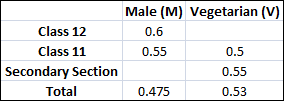### CAT 2007 Question Paper Question 39

Instructions

DIRECTIONS for the following four questions:

The proportion of male students and the proportion of vegetarian students in a school are given below. The school has a total of 800 students, 80% of whom are in the Secondary Section and rest equally divided between Class 11 and 12.Question 39

# In Class 12, twenty five per cent of the vegetarians are male. What is the difference between the number of female vegetarians and male non-vegetarians?

Solution

There are 640 secondary section students, 80 11th class students and 80 12th class students.

If the fraction of vegetarians in 12th class is x, then

80x + 80*0.5 + 640*0.55 = 0.53*800

=> x + 0.5 + 4.4 = 5.3 => x = 0.4

=> There are 32 vegetarian students in class 12.

25% of class 12 i.e 8 are males.

So there are 24 vegetarian female students.

No. of non-veg males = 40 and veg females=24. Required answer 40 - 24 = 16.